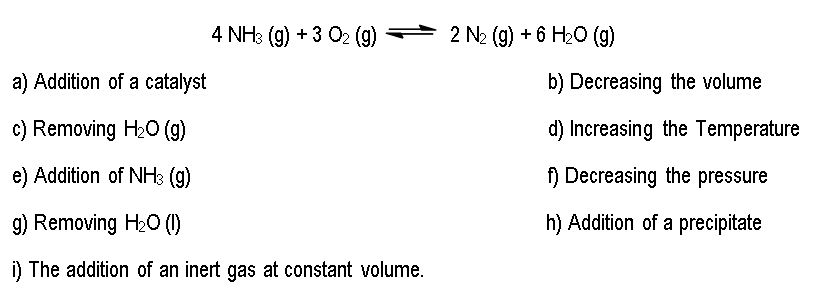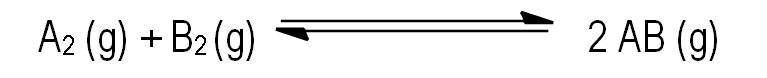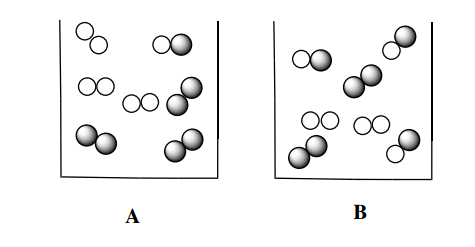Clutch Prep is now a part of Pearson
Ch.7 Energy, Rate and EquilibriumWorksheetSee all chapters

# Le Chatelier's Principle

See all sections
Sections
Nature of Energy
First Law of Thermodynamics
Endothermic & Exothermic Reactions
Bond Energy
Thermochemical Equations
Heat Capacity
Thermal Equilibrium (Simplified)
Hess's Law
Rate of Reaction
Energy Diagrams
Chemical Equilibrium
The Equilibrium Constant
Le Chatelier's Principle
Solubility Product Constant (Ksp)
Spontaneous Reaction
Entropy (Simplified)
Gibbs Free Energy (Simplified)

Le Chatelier's Principle explains what happens to a system at equilibrium when it is disturbed.

###### Thermodynamics and Le Chatelier’s Principle

Concept #1: Understanding Le Chatelier’s Principle.

Transcript

In this video we're going to continue our discussion of chemical equilibria by taking a look at the equilibrium constant and its relationship to thermodynamics. So, here earlier we learn that kinetics or chemical kinetics studied the rate at which our reactants changed into products. So, basically we're looking at the rate or speed of reactions, in this chapter we're now going to learn about thermodynamics and thermodynamics deals with the direction that a chemical reaction at equilibrium will shift. So, thermodynamics deals with the directional reaction takes whereas kinetics looks at the rate or speed at which your reaction has, you need to take a look at both of them to get a complete idea of a chemical reaction. Now, we're going to say here, that Le Chatelier's principle, so Le Chatelier's principle states that state that once a system, which is a chemical reaction, that is at equilibrium is disturbed it will do whatever it can to get back to equilibrium. So, basically you do something to try to alter the equilibrium state and the chemical reaction shifts to the left or to the right in order to minimize that effect. Now, knowing this, let's try to attempt to answer the questions below and look at the different things that we can do to a chemical reaction and as a result of that change, what happens to our chemical reaction.

According to Le Chatelier’s Principle if a system (chemical reaction) is at equilibrium and we disturb it then the system will readjust to maintain it’s equilibrium state.Example #1: The following is an endothermic reaction where Kc = 6.73 x 103.

For each of the choices below predict in which direction the reaction will proceed.

Transcript

If we take a look at this example, we’ll see how exactly does Le Chatelier’s principle help us understand will the reaction shift to the left, to the right or cause no shift at all.
EXAMPLE: For the following endothermic reaction KC = 6.73 x 103. Predict in which direction the reaction will proceed as we do each one of these changes.
4 NH3 (g) + 3 O2 (g) ⇄ 2 N2 (g) + 6 H2O (g)
Now, first things first. We said that this is endothermic so that means that enthalpy, our delta H, value (ΔH) is positive and remember endothermic reactions they absorb heat, and if we’re absorbing heat, heat will be a reactant. Remember, this is the opposite of an exothermic reaction. Exothermic reaction does the complete opposite, it releases heat and therefore heat would be a product, so be aware of that. So here it’s endothermic so ΔH is positive. Now, we’re talking about the reaction shifting to the left or to the right or causing no shift at all.
For A, it says the addition of a catalyst. What we should realize is that catalyst deals with Kinetics. It deals with speed. It lowers the energy of activation in order for the reaction to go faster. So we’re going to say when it comes to Thermodynamics though, a catalyst doesn’t affect the direction of the reaction, so the reaction will not shift to the left of to the right because catalyst deal with Kinetics. Le Chatelier’s principle deals with Thermodynamics.
Next, we’re going to say decreasing the volume. What we should realize here is volume is the opposite of pressure, so if we’re decreasing the volume, we’re increasing the pressure, and in both cases, reaction shifts to side with less moles of gas. So let’s take a look. Here in our equation we have:
4 NH3 (g) + 3 O2 (g) ⇄ 2 N2 (g) + 6 H2O (g)
7 moles of gas 8 moles of gas
So it’s going to the side with less moles of gas, so it’s going to the shift to the left or as we say it’s going to go in the reverse direction. That’s what we would say for B.
For C, we’re removing H2O gas. Now, we’re going to say H2O gas is a product. So here we’re going to say adding reactant or removing product causes the reaction to shift in the forward direction, so it’s going to go to the right because you have to think of this in this sense, we’re at equilibrium so we’re balance, we’re at peace with ourselves as a reaction, then I come in and I remove some product. Now, we have to get back to this balance that we had in the beginning, so if I’ll remove something I have to go towards it to make more of it. So if I’m removing products, how can I remake the product that I just lost? I have to go into forward direction. Going in the forward direction would mean I’m producing more products.
In the same way, if I add reactant, oh, I have too much reactant, I have to get that amount back down to what it was before, so how do I get the reactant amounts down? I’m also going to forward direction because going into forward direction, our reactants would be breaking down in order to build up our products, and just realize for b and c, if I’m saying that I make shifts to the side with less moles when volume is decreased and pressure is increased, then what would happen if I did the reverse?
What would happen if I increase the volume and decrease the pressure? We would expect the opposite to occur and same thing here let’s say we were removing reactant or adding product then you would expect the opposite occur. So remember, what I’m telling you one way, think of it the opposite way also.
Now D is incredibly important. Here, we’re talking about temperature. It was important that we identified that the reaction was endothermic because endothermic/exothermic reactions deal with heat and temperature and heat are very close together. They’re not the same thing but they’re related together.
So we’re going to say increasing the temperature, reaction shifts away from heat that’s why it’s important for us to identify what side the heat was on, was it a reactant or was it a product, and to know this we have to figure out what do endothermic mean. So we’re going to shift away from the heat. The heat is a reactant so we’re going to move away from it. So we’re going to go the right direction, so we’re going to go on the forward direction.
So in the opposite way, if I decrease my temperature, my reaction would shift towards my heat. So remember if it’s true one way, it’s the opposite way.
Adding NH3 (g). So NH3 (g) is a reactant and we just said that adding reactant, it will go in the forward direction.
Next one is decreasing the pressure which is the same thing as increasing volume. Now here, remember, so in this case, reaction shifts to side with more moles of gas. It’s incredibly important you figure out which side has more moles of gas, we said this earlier that the product side happens to have 8 moles so it has most moles of gas. That’s why we’re going into the forward direction.
Let’s say your professor gave you a different equation where moles-to-moles was reactants. Well that would mean in that case, that your reaction would be going in the reverse direction because when you’re decreasing your pressure or increasing your volume, your reaction must shift to the side which more moles of gas whether that be the side with the products or the side with reactants.
Here, we’re removing water liquid. Now, what have we been saying this whole time? We’re dealing with Equilibrium constants; we’re dealing with ice charts. We’ve been saying this whole time what two phases do we ignore. We ignore solids and we ignore liquids, so adding or removing them does nothing to shift the reaction. Solids and liquids cause no shift, so we’ve ignored them in the equilibrium constant, we’ve ignored them in the ice charts, and we’re ignoring them now in the Le Chatelier’s principle.
Next, addition of a precipitate. Remember, what’s a precipitate? Well, it’s a fancy way of saying a solid, so we’re adding a solid. So this would the same exact answer here as well. Remember, solids and liquids cause no shift.
Finally, the addition of an inert gas at a constant volume. Now an inert gas is what? Inert gas is a noble gas and we’re assuming if we’re adding inert gas to our sample that the volume is going to be held constant and that’s because most of the noble gases are unreactive. So as a result of that, we’re also going to say that adding inert gas or noble gases also causes no shift in our reaction.
So again we’re going to say adding a catalyst, adding a solid, adding a liquid, adding a noble gas which we assume will be at a constant volume then we’re going to say there is no shift in the reaction and as long as you can remember what’s going to happen when I mess around with the temperature, when I play around with the pressure and volume and when I add or remove products and reactants which way will it shift.
These are all the concepts that you really need to know when it comes to Le Chatelier’s principle. It’s quite a few but as long as you just remember the basic principles, you’ll be able to answer any type of Le Chatelier’s question you’ll see on the exam.

Practice: Consider the reaction below:

CH4 (g)  +  F2 (g)  ⇌  CF4 (g)  + HF (g)   H = + 38.1 KJ/mol

The following changes will shift the equilibrium to the left except one. Which one would not cause a shift to the left?

Practice: The following data was collected for the following reaction at equilibrium

2 A (g) + 3 B (g)  ⇌ C (g)

At 25 oC K is 5.2 x 10-4 and at 50 oC K is 1.7 x 10-7. Which of the following statements is true?

a) The reaction is exothermic.

b) The reaction is endothermic.

c) The enthalpy change, ΔH, is equal to zero.

d) Not enough information is given.

When looking at a chemical reaction we look at the two concepts of Kinetics and Thermodynamics to get a total view. However, remember that Le Chatelier’s Principle deals with Thermodynamics and not Kinetics.

Practice: Which direction will the following reaction (in a 10.0 L flask) proceed if a catalyst is added to the system?

CaCO3 (s)  ⇌ CaO (s) + CO2 (g)            Kp = 3.2 x 10-28Practice: Consider the following gas reaction of A2 ( shaded spheres) and B2 ( unshaded spheres)

A2 (g)  +  B(g)  ⇌  2 AB (g)

Which container proceeds more to completion?